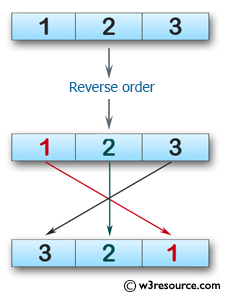﻿ Swift Array Programming Exercise: Create a new array with the elements in reverse order of a given array of integers - w3resource# Swift Array Programming Exercises: Create a new array with the elements in reverse order of a given array of integers

## Swift Array Programming: Exercise-6 with Solution

Write a Swift program to create a new array with the elements in reverse order of a given array of integers .

/*Given an array of ints length 3, return a new array with the elements in reverse order, so {1, 2, 3} becomes {3, 2, 1}.*/

Pictorial Presentation:Sample Solution:

Swift Code:

``````import Foundation
func reverse3(_ nums: [Int]) -> [Int] {
return [nums, nums, nums]
}
print(reverse3([1, 2, 3]))
print(reverse3([5, 11, 9]))
print(reverse3([7, 0, 0]))
print(reverse3([2, 1, 2]))
print(reverse3([1, 2, 1]))
print(reverse3([2, 11, 3]))
print(reverse3([0, 6, 5]))
print(reverse3([7, 2, 3]))
```
```

Sample Output:

```[3, 2, 1]
[9, 11, 5]
[0, 0, 7]
[2, 1, 2]
[1, 2, 1]
[3, 11, 2]
[5, 6, 0]
[3, 2, 7]
```

Swift Programming Code Editor:

Improve this sample solution and post your code through Disqus

What is the difficulty level of this exercise?

﻿